• 构造平衡二叉树 期末题一： 对关键字序列{23，76，47，53，41，12，85，30，90，100}，构造一棵平衡二叉树并画图。 按照顺序依次插入，第一数是23，作为根节点。 第二个是76，比23大，作为23的右孩子。 第三个是47...
构造平衡二叉树
期末题一：
对关键字序列{23，76，47，53，41，12，85，30，90，100}，构造一棵平衡二叉树并画图。
按照顺序依次插入，第一数是23，作为根节点。
第二个是76，比23大，作为23的右孩子。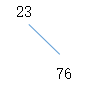第三个是47，大于23所以放在23右侧，再看23的右侧是76，47比76小，所以作为76的左孩子。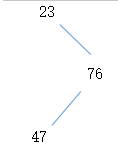到这一步时，计算每个节点的值发现不平衡了。23右边有两层节点，左边无，两边之差为2，不是0或者-1或者1.所以要调整节点到平衡状态。将47调整为根节点，23为左孩子，76为右孩子，重新达到平衡。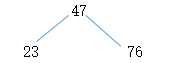然后继续加节点，下一个是53，大于47看右侧，小于76，作为76的左孩子。
下一个是41，小于47看左，大于23，作为23右孩子。以此类推。。。发现没有插入节点后导致树不平衡的状态，所以不需要再调整，结束。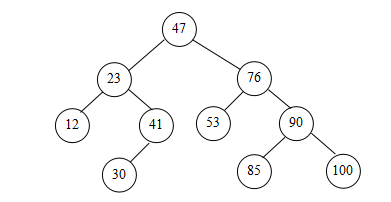qq交流群：979552163


展开全文数据结构
• 什么是平衡二叉树 平衡二叉树，又称为AVL树。它或者是空树，或者是具有下列性质的二叉树：它的左子树和右子树都是平衡二叉树，且左子树和右子树的深度之差的...假设表中关键字序列（13，24，37，90，53） 基本性...
什么是平衡二叉树
平衡二叉树，又称为AVL树。它或者是空树，或者是具有下列性质的二叉树：它的左子树和右子树都是平衡二叉树，且左子树和右子树的深度之差的绝对值不超过1。
平衡二叉树的平衡旋转

LL型。单向右旋平衡处理。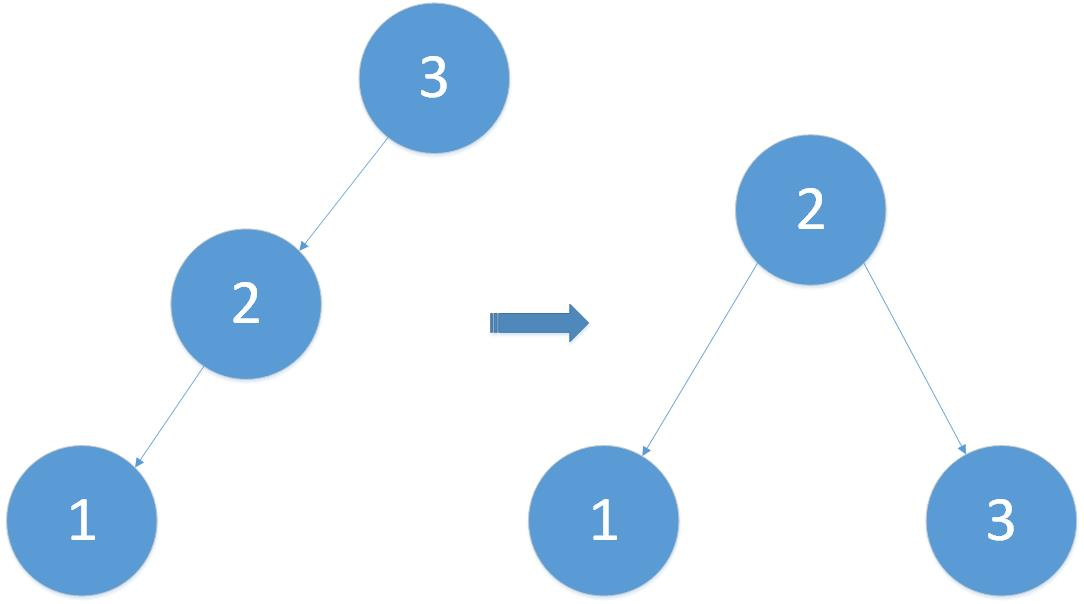2. RR型。单向左旋平衡处理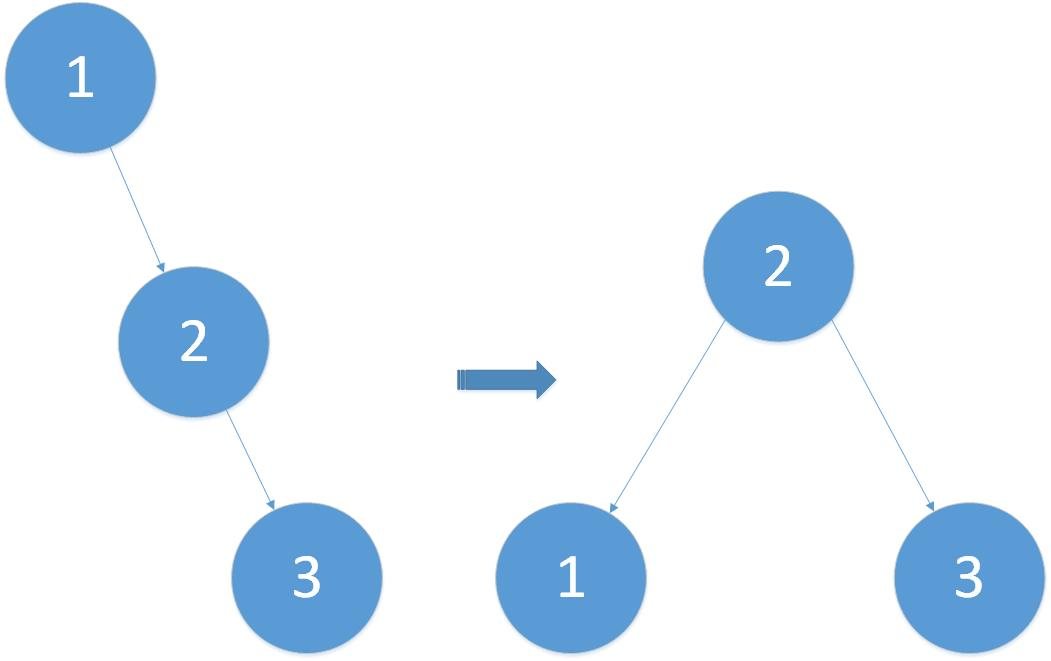3. LR型。双向旋转（先左后右）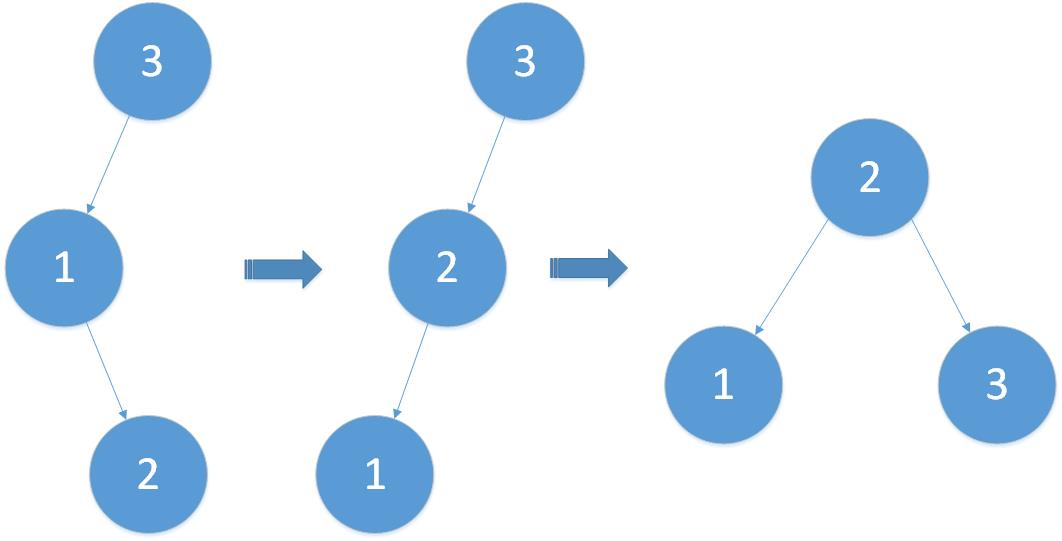４.　RL型。双向旋转（先右后左）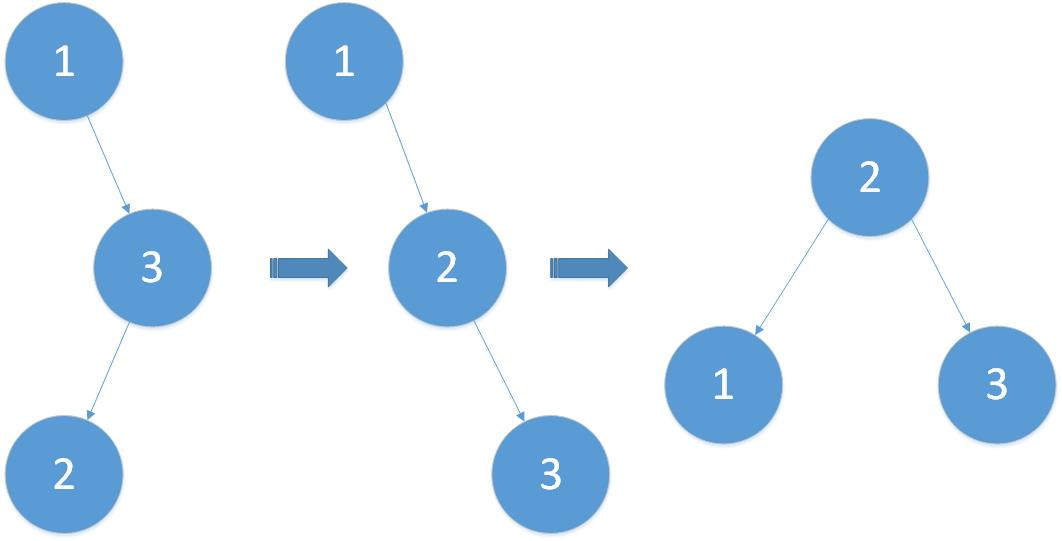构造AVL
假设表中关键字序列（13，24，37，90，53）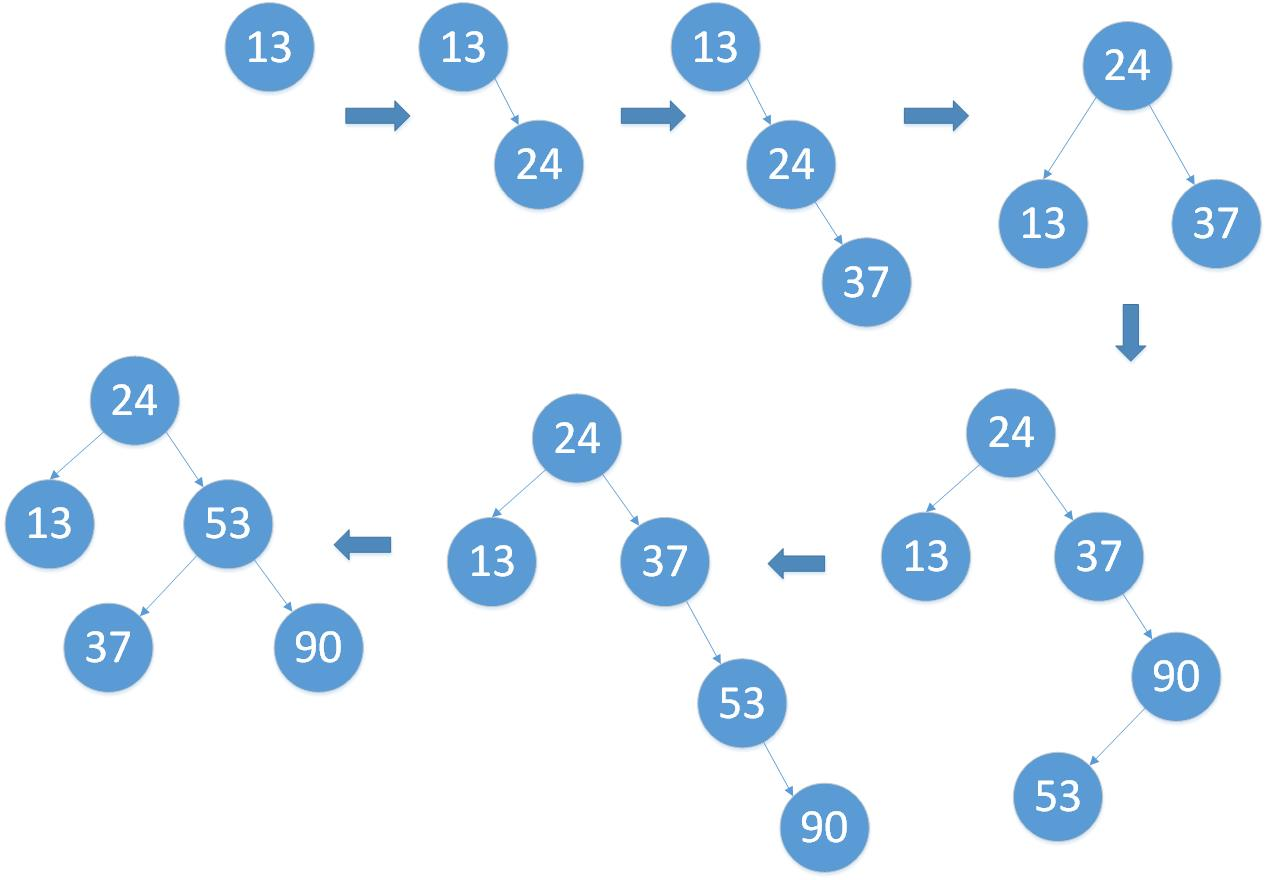基本性质

平衡二叉树进行查找的时间复杂度：O(logn)
深度为h的平衡二叉树的最小结点：Nh = N(h-1) + N(h-2) + 1


展开全文数据结构
• （2）输入一个关键字序列，建立对应的平衡二叉树； （3）向平衡二叉树中插入一个结点（关键字）； （4）从平衡二叉树中删除一个结点（关键字）； （5）计算平衡二叉树的平均查找长度； （6）销毁平衡二叉树。 概要...
需求分析
实现平衡二叉树的创建，插入，删除，查询操作。并求出所构建平衡二叉排序树的平均查找长度ASL。
（1）以二叉链表作为平衡二叉树的存储结构；
（2）输入一个关键字序列，建立对应的平衡二叉树；
（3）向平衡二叉树中插入一个结点（关键字）；
（4）从平衡二叉树中删除一个结点（关键字）；
（5）计算平衡二叉树的平均查找长度；
（6）销毁平衡二叉树。
概要设计
平衡二叉树是在构造二叉排序树的过程中，每当插入一个新结点时，首先检查是否因插入新结点而破坏了二叉排序树的平衡性，若是则找出其中的最小不平衡子树，在保持二叉排序树特性的前提下，调整最小不平衡子树中各结点之间的链接关系，进行相应的旋转，使之成为新的平衡子树。
详细设计
#include<stdio.h>
#include<stdlib.h>
#include<math.h>
#define TRUE 1
#define FALSE 0
#define LH    1       //左高
#define EH    0       //一样高
#define RH   -1       //右高
typedef int Status;
typedef int ElemType;
typedef bool Boolean;   //(bool型变量就是逻辑变量的定义符,布尔型变量的值只有真或假，而Boolean类型的变量是一个无符号的char变量)

typedef struct BSTNode//二叉树的二叉链表定义
{
ElemType data;
int bf;       //结点的平衡因子
struct BSTNode *lchild, *rchild; //左，右孩子指针
}BSTNode, *BSTree;

void R_Rotate(BSTree &p)  //以*p为根的二叉排序做右旋处理
{
BSTree lc = p->lchild; //lc指向*p左子树根结点
p->lchild = lc->rchild; //lc右子树挂接为*p左子树
lc->rchild = p; //p指向新的根结点
p = lc;
}//R_Rotate

//以*p为根的二叉排序做左旋处理
void L_Rotate(BSTree &p)
{
BSTree rc = p->rchild; //rc指向*p右子树根结点
p->rchild = rc->lchild; //rc左子树挂接为*p右子树
rc->lchild = p; //p指向新的根结点
p = rc;
}//L_Rotate
// 对二叉树T进行左平衡处理(LL型和LR型)
void LeftBalance(BSTree &T)
{
BSTree lc = T->lchild;
switch (lc->bf)
{
//LL型的只需要进行右旋操作
case LH:

//右旋之后根和左子树都的平衡的
T->bf = lc->bf = EH;
R_Rotate(T); //右旋操作
break;
//LR型的需要进行左旋操作，然后右旋操作
case RH:
BSTree rd = lc->rchild;
switch (rd->bf)
{ //修改*T及其左孩子的平衡因子
case LH:T->bf = RH; lc->bf = EH; break;
case EH:T->bf = EH; lc->bf = EH; break;
case RH:T->bf = EH; lc->bf = LH; break;
} //switch (rd->bf)
rd->bf = EH;
L_Rotate(T->lchild);//对*T左子树作左旋平衡处理
R_Rotate(T);//对*T作右旋平衡处理
break;
} //switch (lc->bf)
} //LeftBalance

void RightBalance(BSTree & T)

{
BSTree rc = T->rchild;
switch (rc->bf)
{
//RR型只需要做左旋操作
case RH:
T->bf = EH;
rc->bf = EH;
L_Rotate(T); //左旋操作
break;
//RL型需要先做右旋操作，然后做左旋操作
case LH:
BSTree ld = rc->lchild;
switch (ld->bf)
{
case LH:T->bf = EH; rc->bf = RH; break;
case EH:T->bf = EH; rc->bf = EH; break;
case RH:T->bf = LH; rc->bf = EH; break;
} //switch (ld->bf)
ld->bf = EH;
R_Rotate(T->rchild);
L_Rotate(T);
break;
}//switch (rc->bf)
} //RightBalance

//把元素e插入平衡二叉树T中

Status InsertAVL(BSTree &T, int e, Boolean & taller)
{
if (!T)//插入新节点，树长高，置taller为TRUE
{
T = (BSTree)malloc(sizeof(BSTNode));
T->rchild = NULL;
T->lchild = NULL;
T->data = e;
T->bf = EH;
taller = TRUE;
}
else
{
//该元素已经在平衡二叉树中存在了
if (e == T->data)
{
taller = FALSE;
return FALSE;
}
//插入左子树
else if (e < T->data)
{
if (!InsertAVL(T->lchild, e, taller)) return FALSE; //未插入
if (taller) //插入成功，并且树变高了
{
switch (T->bf)
{
case LH:
LeftBalance(T); taller = FALSE; break;
//平衡二叉树做完左平衡操作后
//树高没有变化，故taller = false
case EH:
T->bf = LH; taller = TRUE; break;
//原来是平衡的故插入一个元素后
//树高必然变高
case RH:
T->bf = EH; taller = FALSE; break;
//原来是右子树比左子树高，但是当向左子树中
//插入一个元素的时候，树变平衡了，故taller = false
} //switch (T->bf)
} //if (taller)
}
//插入右子树
else
{
if (!InsertAVL(T->rchild, e, taller)) return 0; //未插入
if (taller)
{
switch (T->bf)
{
case LH:
T->bf = EH;
taller = FALSE;
break;
case EH:
T->bf = RH;
taller = TRUE;
break;
case RH:
RightBalance(T);
taller = FALSE;
break;
} // switch (T->bf)
} //if (taller)
} //else
} //else
return TRUE;
}//InsertAVL

//从平衡二叉树中删除一个结点（关键字）
Status DeleteAVL(BSTree &T, int key, Boolean &shorter)
{
if (!T)
{//没有关键字
shorter = FALSE;
printf("该平衡二叉树中无此关键字！\n");
return 0;
}
else
{
if (key == T->data) //找到了需要删除的结点
{
//如果该结点的lchild 和
//rchild 至少有一个为NULL
//则大功告成，否则请参照
//下方解释
BSTree q = T;
if (!T->lchild)//如果该结点的lchild 为NULL
{
q = T;
T = T->rchild;
free(q);
shorter = TRUE;
return TRUE;
}
else if (!T->rchild) {//如果该结点的rchild 为 NULL
q = T;
T = T->lchild;//如果不是&（引用）的强大功能，这句话是没有意义的
free(q);
shorter = TRUE;
return TRUE;
}
else {
//删除一个左右孩子都不为空的结点
//使该结点的直接前驱p的data替换该结点的data
//然后改变key=p.data
BSTree s = T->lchild;
while (s->rchild)
s = s->rchild;
T->data = s->data;
key = s->data;
}
}
if (key < T->data)
{
if (!DeleteAVL(T->lchild, key, shorter)) return 0;
if (shorter)
{
switch (T->bf)
{
case LH:T->bf = EH; shorter = TRUE; break;
case EH:T->bf = RH; shorter = FALSE; break;
case RH:RightBalance(T);
if (T->rchild->bf == EH)
shorter = FALSE;
else
shorter = TRUE; break;
}//switch(T->bf)
}
}
else {
if (!DeleteAVL(T->rchild, key, shorter)) return 0;
if (shorter)
{
switch (T->bf)
{
case LH:LeftBalance(T);
if (T->lchild->bf == EH)
shorter = FALSE;
else
shorter = TRUE; break;
case EH:T->bf = LH; shorter = FALSE; break;
case RH:T->bf = EH; shorter = TRUE; break;
}
}
}
}
return 1;
}//DeleteAVL
//计算平衡二叉树的平均查找长度(查找关键字e)
Status SearchBST(BSTree & T, int e)
{
if (T == NULL)
{
printf("该平衡二叉树中无此关键字！\n");
return NULL;
}
if (T->data == e)
{
return T->data;
}
else if (e < T->data)
{
return SearchBST(T->lchild, e);
}
else
{
return SearchBST(T->rchild, e);
}
}
//销毁平衡二叉树
void DestroyBST(BSTree & T)
{
if (NULL == T) return;
DestroyBST(T->lchild);
DestroyBST(T->rchild);
free(T);
}
//输出平衡二叉树中的所有的元素（小->大，中序遍历）
void PrintBST(BSTree & T)
{
if (NULL == T) return;
PrintBST(T->lchild);
printf("%d ", T->data);
PrintBST(T->rchild);
}
//7-统计树的叶子结点个数
Status CountLeafs(BSTree T)
{
int i, j;
if (T)
{
i = CountLeafs(T->lchild);
j = CountLeafs(T->rchild);
return i + j + 1;
}
else
return 0;
}
//平均查找长度
float ASL(BSTree T)
{
float n;
float ALS;
n = CountLeafs(T);
ALS = ((n + 1) / n)*(log10(n + 1) / log10(2)) - 1;
return ALS;
}

int main()
{
BSTree T = NULL;
bool taller = false, shorter;
int n; float c;
printf("请输入数据 ，输入0结束:\n");
while (scanf_s("%d", &n))
{
if (n == 0) break;
else InsertAVL(T, n, taller);
}
printf("平衡二叉树建立成功！\n");
printf("请输入插入的结点:");
scanf_s("%d", &n);
InsertAVL(T, n, taller);
printf("插入成功！\n");
printf("请输入需要删除的结点:");
scanf_s("%d", &n);
DeleteAVL(T, n, shorter);
c = ASL(T);
printf("平均查找长度为:\n");
printf("ASL=%5.2f\n", c);
printf("其结果为：\n");
PrintBST(T);
printf("\n");
DestroyBST(T);
system("pause");
}


测试与结果展开全文数据结构
• 回顾二叉排序树的查找性能 若查找...关键字序列为(45,24,53,12,37,93)所构造的二叉排序树如图(a)所示 回顾二叉排序树的形态(续) 如果根据关键字的输入序列构造二叉树为单枝树则其平均查找长度与顺序查找相同因此在
• 问题描述：利用平衡二叉树对序列...程序输出：构造平衡二叉树、对平衡二叉树进行删除 。 #include #include typedef int KeyType; //定义关键字类型 typedef char InfoType; typedef struct node //记录类型
问题描述：利用平衡二叉树对序列排序后进行查找和删除 。
程序输出：构造平衡二叉树、对平衡二叉树进行删除 。
#include <stdio.h>
#include <malloc.h>
typedef int KeyType;                    //定义关键字类型
typedef char InfoType;
typedef struct node                     //记录类型
{
KeyType key;                        //关键字项
int bf;                             //平衡因子
InfoType data;                      //其他数据域
struct node *lchild,*rchild;        //左右孩子指针
} BSTNode;
void LeftProcess(BSTNode *&p,int &taller)
//对以指针p所指结点为根的二叉树作左平衡旋转处理,本算法结束时,指针p指向新的根结点
{
BSTNode *p1,*p2;
if (p->bf==0)           //原本左、右子树等高,现因左子树增高而使树增高
{
p->bf=1;
taller=1;
}
else if (p->bf==-1)     //原本右子树比左子树高,现左、右子树等高
{
p->bf=0;
taller=0;
}
else                    //原本左子树比右子树高,需作左子树的平衡处理
{
p1=p->lchild;       //p指向*p的左子树根结点
if (p1->bf==1)      //新结点插入在*b的左孩子的左子树上,要作LL调整
{
p->lchild=p1->rchild;
p1->rchild=p;
p->bf=p1->bf=0;
p=p1;
}
else if (p1->bf==-1)    //新结点插入在*b的左孩子的右子树上,要作LR调整
{
p2=p1->rchild;
p1->rchild=p2->lchild;
p2->lchild=p1;
p->lchild=p2->rchild;
p2->rchild=p;
if (p2->bf==0)          //新结点插在*p2处作为叶子结点的情况
p->bf=p1->bf=0;
else if (p2->bf==1)     //新结点插在*p2的左子树上的情况
{
p1->bf=0;
p->bf=-1;
}
else                    //新结点插在*p2的右子树上的情况
{
p1->bf=1;
p->bf=0;
}
p=p2;
p->bf=0;            //仍将p指向新的根结点,并置其bf值为0
}
taller=0;
}
}
void RightProcess(BSTNode *&p,int &taller)
//对以指针p所指结点为根的二叉树作右平衡旋转处理,本算法结束时,指针p指向新的根结点
{
BSTNode *p1,*p2;
if (p->bf==0)           //原本左、右子树等高,现因右子树增高而使树增高
{
p->bf=-1;
taller=1;
}
else if (p->bf==1)      //原本左子树比右子树高,现左、右子树等高
{
p->bf=0;
taller=0;
}
else                    //原本右子树比左子树高,需作右子树的平衡处理
{
p1=p->rchild;       //p指向*p的右子树根结点
if (p1->bf==-1)     //新结点插入在*b的右孩子的右子树上,要作RR调整
{
p->rchild=p1->lchild;
p1->lchild=p;
p->bf=p1->bf=0;
p=p1;
}
else if (p1->bf==1) //新结点插入在*p的右孩子的左子树上,要作RL调整
{
p2=p1->lchild;
p1->lchild=p2->rchild;
p2->rchild=p1;
p->rchild=p2->lchild;
p2->lchild=p;
if (p2->bf==0)          //新结点插在*p2处作为叶子结点的情况
p->bf=p1->bf=0;
else if (p2->bf==-1)    //新结点插在*p2的右子树上的情况
{
p1->bf=0;
p->bf=1;
}
else                    //新结点插在*p2的左子树上的情况
{
p1->bf=-1;
p->bf=0;
}
p=p2;
p->bf=0;            //仍将p指向新的根结点,并置其bf值为0
}
taller=0;
}
}
int InsertAVL(BSTNode *&b,KeyType e,int &taller)
/*若在平衡的二叉排序树b中不存在和e有相同关键字的结点,则插入一个
数据元素为e的新结点,并返回1,否则返回0。若因插入而使二叉排序树
失去平衡,则作平衡旋转处理,布尔变量taller反映b长高与否*/
{
if(b==NULL)         //原为空树,插入新结点,树“长高”,置taller为1
{
b=(BSTNode *)malloc(sizeof(BSTNode));
b->key=e;
b->lchild=b->rchild=NULL;
b->bf=0;
taller=1;
}
else
{
if (e==b->key)              //树中已存在和e有相同关键字的结点则不再插入
{
taller=0;
return 0;
}
if (e<b->key)               //应继续在*b的左子树中进行搜索
{
if ((InsertAVL(b->lchild,e,taller))==0) //未插入
return 0;
if (taller==1)          //已插入到*b的左子树中且左子树“长高”
LeftProcess(b,taller);
}
else                        //应继续在*b的右子树中进行搜索
{
if ((InsertAVL(b->rchild,e,taller))==0) //未插入
return 0;
if (taller==1)          //已插入到b的右子树且右子树“长高”
RightProcess(b,taller);
}
}
return 1;
}
void DispBSTree(BSTNode *b) //以括号表示法输出AVL
{
if (b!=NULL)
{
printf("%d",b->key);
if (b->lchild!=NULL || b->rchild!=NULL)
{
printf("(");
DispBSTree(b->lchild);
if (b->rchild!=NULL) printf(",");
DispBSTree(b->rchild);
printf(")");
}
}
}
void LeftProcess1(BSTNode *&p,int &taller)  //在删除结点时进行左处理
{
BSTNode *p1,*p2;
if (p->bf==1)
{
p->bf=0;
taller=1;
}
else if (p->bf==0)
{
p->bf=-1;
taller=0;
}
else        //p->bf=-1
{
p1=p->rchild;
if (p1->bf==0)          //需作RR调整
{
p->rchild=p1->lchild;
p1->lchild=p;
p1->bf=1;
p->bf=-1;
p=p1;
taller=0;
}
else if (p1->bf==-1)    //需作RR调整
{
p->rchild=p1->lchild;
p1->lchild=p;
p->bf=p1->bf=0;
p=p1;
taller=1;
}
else                    //需作RL调整
{
p2=p1->lchild;
p1->lchild=p2->rchild;
p2->rchild=p1;
p->rchild=p2->lchild;
p2->lchild=p;
if (p2->bf==0)
{
p->bf=0;
p1->bf=0;
}
else if (p2->bf==-1)
{
p->bf=1;
p1->bf=0;
}
else
{
p->bf=0;
p1->bf=-1;
}
p2->bf=0;
p=p2;
taller=1;
}
}
}
void RightProcess1(BSTNode *&p,int &taller) //在删除结点时进行右处理
{
BSTNode *p1,*p2;
if (p->bf==-1)
{
p->bf=0;
taller=-1;
}
else if (p->bf==0)
{
p->bf=1;
taller=0;
}
else        //p->bf=1
{
p1=p->lchild;
if (p1->bf==0)          //需作LL调整
{
p->lchild=p1->rchild;
p1->rchild=p;
p1->bf=-1;
p->bf=1;
p=p1;
taller=0;
}
else if (p1->bf==1)     //需作LL调整
{
p->lchild=p1->rchild;
p1->rchild=p;
p->bf=p1->bf=0;
p=p1;
taller=1;
}
else                    //需作LR调整
{
p2=p1->rchild;
p1->rchild=p2->lchild;
p2->lchild=p1;
p->lchild=p2->rchild;
p2->rchild=p;
if (p2->bf==0)
{
p->bf=0;
p1->bf=0;
}
else if (p2->bf==1)
{
p->bf=-1;
p1->bf=0;
}
else
{
p->bf=0;
p1->bf=1;
}
p2->bf=0;
p=p2;
taller=1;
}
}
}
void Delete2(BSTNode *q,BSTNode *&r,int &taller)
//由DeleteAVL()调用,用于处理被删结点左右子树均不空的情况
{
if (r->rchild==NULL)
{
q->key=r->key;
q=r;
r=r->lchild;
free(q);
taller=1;
}
else
{
Delete2(q,r->rchild,taller);
if (taller==1)
RightProcess1(r,taller);
}
}
int DeleteAVL(BSTNode *&p,KeyType x,int &taller) //在AVL树p中删除关键字为x的结点
{
int k;
BSTNode *q;
if (p==NULL)
return 0;
else if (x<p->key)
{
k=DeleteAVL(p->lchild,x,taller);
if (taller==1)
LeftProcess1(p,taller);
return k;
}
else if (x>p->key)
{
k=DeleteAVL(p->rchild,x,taller);
if (taller==1)
RightProcess1(p,taller);
return k;
}
else            //找到了关键字为x的结点,由p指向它
{
q=p;
if (p->rchild==NULL)        //被删结点右子树为空
{
p=p->lchild;
free(q);
taller=1;
}
else if (p->lchild==NULL)   //被删结点左子树为空
{
p=p->rchild;
free(q);
taller=1;
}
else                        //被删结点左右子树均不空
{
Delete2(q,q->lchild,taller);
if (taller==1)
LeftProcess1(q,taller);
p=q;
}
return 1;
}
}
int main()
{
BSTNode *b=NULL;
int i,j,k;
KeyType a[]= {16,3,7,11,9,26,18,14,15},n=9; //例10.5
printf(" 创建一棵AVL树:\n");
for(i=0; i<n; i++)
{
printf("   第%d步,插入%d元素:",i+1,a[i]);
InsertAVL(b,a[i],j);
DispBSTree(b);
printf("\n");
}
printf("   AVL:");
DispBSTree(b);
printf("\n");
printf(" 删除结点:\n");                     //例10.6
k=11;
printf("   删除结点%d:",k);
DeleteAVL(b,k,j);
printf("   AVL:");
DispBSTree(b);
printf("\n");
k=9;
printf("   删除结点%d:",k);
DeleteAVL(b,k,j);
printf("   AVL:");
DispBSTree(b);
printf("\n");
k=15;
printf("   删除结点%d:",k);
DeleteAVL(b,k,j);
printf("   AVL:");
DispBSTree(b);
printf("\n\n");
return 0;
}

运行结果：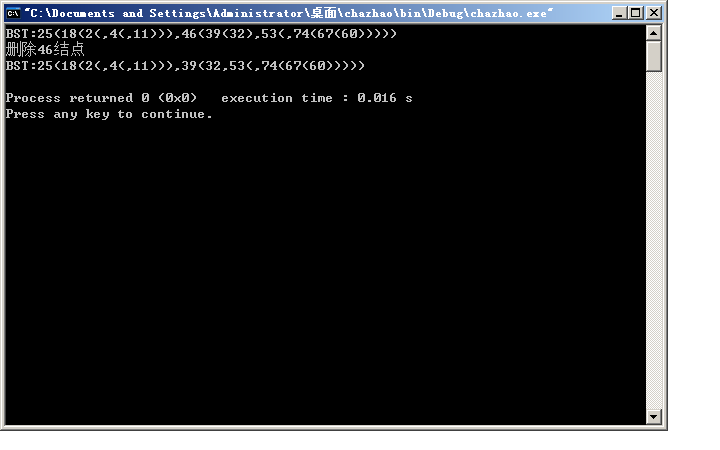展开全文• 上篇博客主要讲述了二叉排序树的基本概念和插入删除操作，必须再次说明的是：在一...对于给定的关键字序列，如何构造一棵形态匀称的二叉排序树。这种匀称的二叉排序树就称为平衡二叉树平衡二叉树定义：平衡二叉树插入
• 构造二叉排序树时，如果输入的数据元素序列恰巧按其关键字大小有序，则在形式上已经退化成一个单链表了。这样查找操作所需要的时间就是O(n),或者说与结点个数成线性关系。 因此需要有一种方法来避免使二叉排序树变...数据结构
• 　平衡二叉树是在构造二叉排序树的过程中，每当插入一个新结点时，首先检查是否因插入新结点而破坏了二叉排序树的平衡性，若是，则找出其中的最小不平衡子树，在保持二叉排序树特性的前提下，调整最小不平衡子树中各...
• *问题描述：认真阅读并验证平衡二叉树相关算法。 （1）由整数序列{43,52,75,24,10,38,67,55,63,60}构造AVL树； （2）输出用括号法表示的AVL树； （3）查找关键字55； （4）分别删除43和55，输出删除后用括号...
• *问题描述：认真阅读并验证平衡二叉树相关算法。 （1）由整数序列{43,52,75,24,10,38,67,55,63,60}构造AVL树； （2）输出用括号法表示的AVL树； （3）查找关键字55； （4）分别删除43和55，输出删除后用...
• /* *2015 ,烟台大学计算机控制工程学院 ...*问题描述：认真阅读并验证平衡二叉树相关算法。 *（1）由整数序列{43,52,75,24,10,38,67,55,63,60}构造AVL树； *（2）输出用括号法表示的AVL树； *（3）查找关键字55；
• *问题描述：认真阅读并验证平衡二叉树相关算法。 （1）由整数序列{43,52,75,24,10,38,67,55,63,60}构造AVL树； （2）输出用括号法表示的AVL树； （3）查找关键字55； （4）分别删除43和55，输
• *问题描述：认真阅读并验证平衡二叉树相关算法。 （1）由整数序列{43,52,75,24,10,38,67,55,63,60}构造AVL树； （2）输出用括号法表示的AVL树； （3）查找关键字55； （4）分别删除43和55，输出删除后用括号...
• 问题描述：认真阅读并验证平衡二叉树相关算法。 （1）由整数序列{43,52,75,24,10,38,67,55,63,60}构造AVL树； （2）输出用括号法表示的AVL树； （3）查找关键字55； （4）分别删除43和55，输出删除后用括号...c++ c语言
• *问题描述：认真阅读并验证平衡二叉树相关算法。 （1）由整数序列{43,52,75,24,10,38,67,55,63,60}构造AVL树； （2）输出用括号法表示的AVL树； （3）查找关键字55； （4）分别删除43和55，输出删除后用括号...
• *问题描述：认真阅读并验证平衡二叉树相关算法。 （1）由整数序列{43,52,75,24,10,38,67,55,63,60}构造AVL树； （2）输出用括号法表示的AVL树； （3）查找关键字55； （4）分别删除43和55，输出删除后用...
• *问题描述：认真阅读并验证平衡二叉树相关算法。 （1）由整数序列{43,52,75,24,10,38,67,55,63,60}构造AVL树； （2）输出用括号法表示的AVL树； （3）查找关键字55； （4）分别删除43和55，输出删除后用括号...
• 二叉排序树（二叉查找树） 二叉排序树或者是一棵空树，或者是具有下列性质：若左子树不空，则左子树上所有结点的值均小于或...构造一颗二叉排序树的目的，并不是为了排序，而是为了提高查找和插入删除关键字的速度。
• *问题描述：认真阅读并验证平衡二叉树相关算法。 （1）由整数序列{43,52,75,24,10,38,67,55,63,60}构造AVL树； （2）输出用括号法表示的AVL树； （3）查找关键字55； （4）分别删除43和55，输出删除后用括
• 1、二叉排序树二叉排序树又称为二叉查找树。...构造二叉排序树的目的：提高查找和插入删除关键字的速度。 注意：二叉排序树中不允许有重复或相等的key值出现。1）删除操作 当删除的节点为叶子节点时，直接遍历 查找 红黑树 二叉排序树
• 验证算法（平衡二叉树） 项目要求如下： （1）由整数序列{43,52,75,24,10,38,67,55,63,60}构造AVL树；  （2）输出用括号法表示的AVL树；  （3）查找关键字55；  （4）分别删除43和55，输出删除后用括号法表示的...
• （1）由整数序列{43,52,75,24,10,38,67,55,63,60}构造AVL树； （2）输出用括号法表示的AVL树； （3）查找关键字55； （4）分别删除43和55，输出删除后用括号法表示的二叉排序树。 输入描述： 程序输出： ...
• /*  ... * All rights reserved.... * 文件名称:a ...* 问题描述:由整数序列构造二叉排序树，输出括号法表示的二叉排序树，用递归和非递归算法查找关键字，输出删除后用括号法表示的二叉排序树。
• *All right reserved *文件名称：main.cpp ...*问题描述:（1）由整数序列{43,52,75,24,10,38,67,55,63,60}构造AVL树； （2）输出用括号法表示的AVL树； （3）查找关键字55； （4）分别删除43和55，输出
• 二叉树的中序遍历结果为顺序小到大序列； 二叉排序树的目的不是为了排序，而是为了提高查找(有序）、和删除关键字（树型结构）的速度； 特点：左子树<根节点<右子树 1.构造一颗二叉排序树 bool create_BST...二叉排序树
• 注意:列序的方法类似 考点N维数组 遍历 由中序前后序列画树 给出中序后序序列画树类似 树与...在地址空间为0~16的散列区中根据给出的关键字序列构造两个哈希表(Jan,Feb,Mar,Apr,May,June,July,Aug,Sep,Oct,Nov,Dec.
• 前面两篇我们学习实践的二叉查找树和平衡二叉树以及有序数组的二分查找、单链表的逐个查找，都需要进行比较和递归。那么有没有其他的查询技术呢？通过下图可以看到散列表我们还没有涉及，今天我们就来对散列表（哈希...android c++ 算法
• 关键字序列 31254构造一棵二叉排序树; 结论 含有n个结点的二叉排序树的平均查找长度和树的形态有关最坏的情况为单支树这时树的深度为n,其平均查找长度为(n+1)/2最好的情况是二叉排序树的形态与折半查找相同其平均...